## ML Aggarwal Class 7 Solutions for ICSE Maths Chapter 9 Linear Equations and Inequalities Ex 9.1

Solve the following (1 to 9) equations:
Question 1.
(i) 2 (3 – 2x) = 13
(ii) $$\frac { 3 }{ 5 }$$ y – 2 = $$\frac { 7 }{ 10 }$$
Solution:
(i) 2 (3 – 2x) = 13 (Remove group symbol)
6 – 4x = 13 (Transposing 6 to R.H.S.)
-4x = 13 – 6
-4x = 7
x = $$\frac { 7 }{ -4 }$$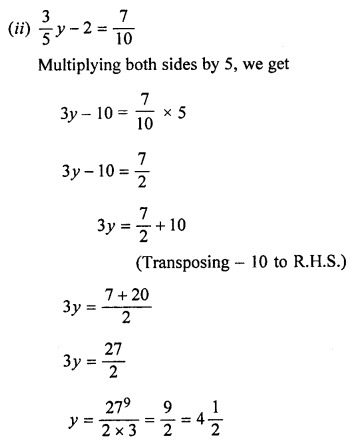Question 2.Solution: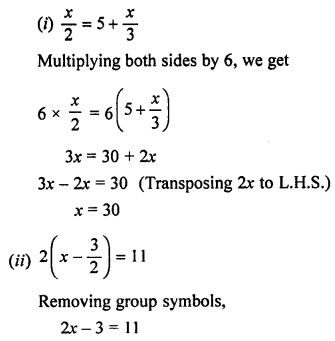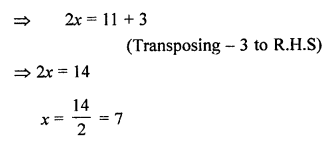Question 3.
(i) 7(x – 2) = 2 (2x – 4)
(ii) 21 – 3(x – 7) = x + 20
Solution:
(i) 7(x – 2) = 2 (2x – 4)
Removing group symbols,
7x – 14 = 4x – 8
7x – 4x = -8 + 14
(Transposing 4x to L.H.S. and -14 to R.H.S.)
3x = 6
x = 2.
(ii) 21 – 3 (x – 7) = x + 20
Removing group symbols,
21 – 3x + 21 = x + 20
⇒ 42 – 3x = x + 20
⇒ -3x – x = 20 – 42
(Transposing x to L.H.S. and 42 to R.H.S.)
⇒ -4x = -22
⇒ x = $$\frac { 22 }{ 4 }$$ = $$\frac { 11 }{ 2 }$$ = 5$$\frac { 1 }{ 2 }$$

Question 4.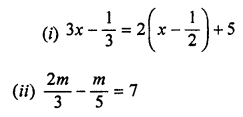Solution: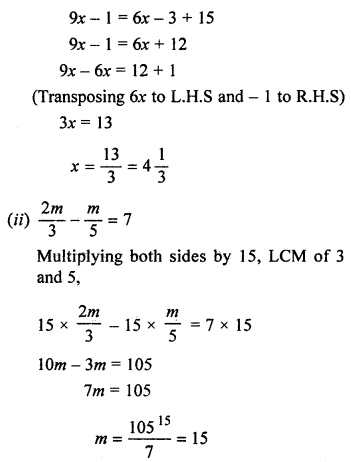Question 5.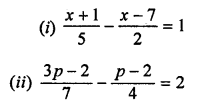Solution: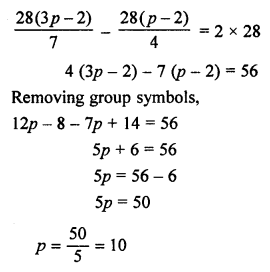Question 6.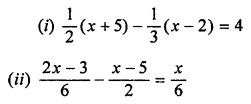Solution: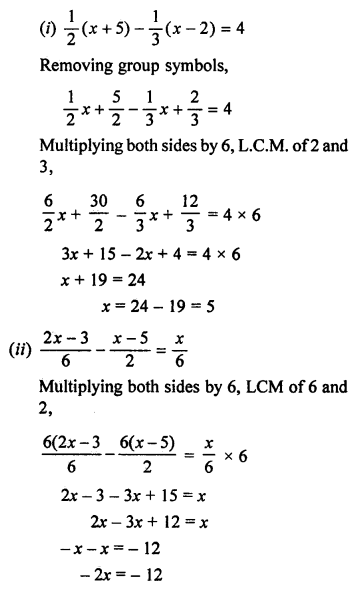x = 6

Question 7.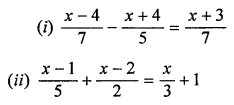Solution: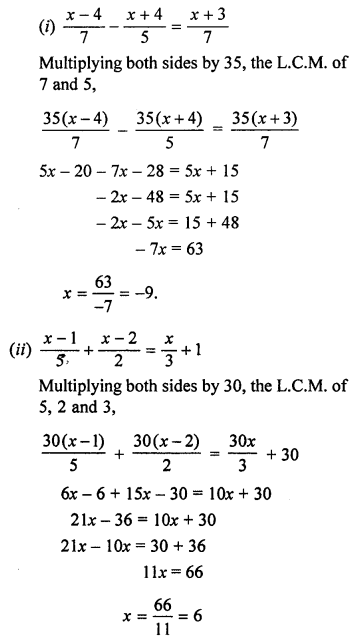Question 8.
(i) y + 1.2y = 4.4
(ii) 15% of x = 21
Solution: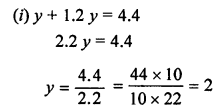Question 9.
(i) 2p + 20% of (2p – 1) = 7
(ii) 3 (2x – 1) + 25% of x = 97
Solution: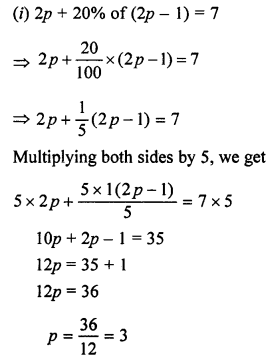Question 10.
Find the value of p if the value of x4 – 3x3 – px – 5 is equal to 23 when x = -2.
Solution:
x = -2
x4 – 3x3 – px – 5 = 23
⇒ (-2)4 – 3(-2)3 – p(-2) – 5 = 23
⇒ 16 + 24 + 2p – 5 = 23
⇒ 35 + 2p = 23
⇒ 2p = 23 – 35 = -12
⇒ p = -6